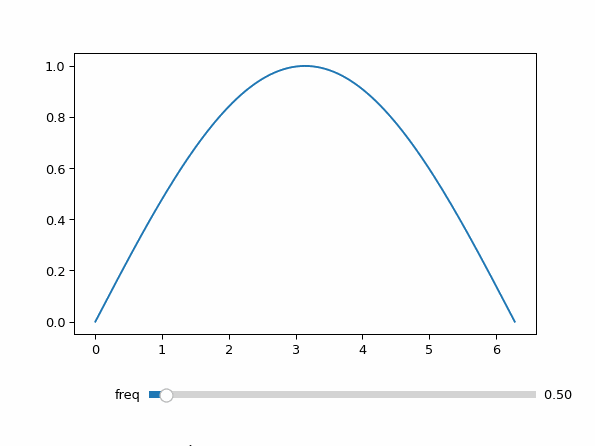# Matplotlib Sliders without a separate Controls Figure¶

Demonstration of how to provide a matplotlib slider to prevent the creation of a separate controls figure.```import matplotlib.pyplot as plt
import numpy as np
from matplotlib.widgets import Slider

from mpl_interactions import ipyplot as iplt

fig, ax = plt.subplots()
x = np.linspace(0, 2 * np.pi, 200)

def f(x, freq):
return np.sin(x * freq)

axfreq = plt.axes([0.25, 0.1, 0.65, 0.03])
slider = Slider(axfreq, label="freq", valmin=0.05, valmax=10)
controls = iplt.plot(x, f, freq=slider, ax=ax)
plt.show()
```

Total running time of the script: ( 0 minutes 8.564 seconds)

Gallery generated by Sphinx-Gallery